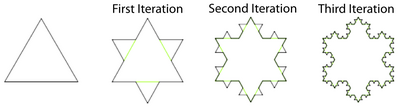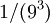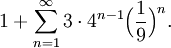# Koch Snowflake Example

Previous: A Geometric Series Problem with Shifting Indicies

Next: Videos on the Introduction to Infinite Series

## Problem

Suppose we would like to calculate the area of the "Koch Snowflake". The Koch Snowflake is an object that can be created from the union of infinitely many equilateral triangles (see figure below).Starting with the equilateral triangle, this diagram gives the first three iterations of the Koch Snowflake (Creative Commons, Wikimedia Commons, 2007).

We construct the Koch Snowflake in an iterative process. Starting with an equilateral triangle, each iteration consists of altering each line segment as follows:

• divide the line segment into three segments of equal length
• draw an equilateral triangle that has the middle segment from step 1 as its base and points outward
• remove the line segment that is the base of the triangle from step 2

The Koch Snowflake is the limit approached as the number of iterations goes to infinity.

## SolutionThe Koch Snowflake after 5 iterations. The first iteration is blue, the second green, the third yellow, the fourth is red, and the fifth is black (Creative Commons, image from Wikimedia Commons).

Now, to derive an expression for the area of our construction at theiteration, let's start with the fifth iteration. The fifth iteration of the snowflake is shown below, with its iterations in different colours.

Blue and Green Triangles

Assume that the one blue triangle as unit area. Each side of the green triangle is exactly 1/3 the length of a side of the blue triangle, and therefore has exactly 1/9 the area of the blue triangle. There are three green triangles, so the green and blue triangles have an area ofBlue, Green, and Yellow Triangles

Each of the 12 = 3•4 yellow triangles has 1/9 the area of a green triangle, orthe area of a blue triangle. The area of the blue, green, and yellow triangles isBlue, Green, Yellow, and Red Triangles

Each of the 48 = 3•4•4 red triangles has 1/9 the area of a yellow triangles, orthe area of a blue triangle. The area of the blue, green, yellow, and red triangles isTotal Area

The total area of the snowflake uses the infinite sequence.

We will add all the terms of the series together, and add 1, to produce the following sumSeeing that this is a geometric series with a = 1/3 and r = 4/9, we immediately conclude that this series converges and is equal toPrevious: A Geometric Series Problem with Shifting Indicies

Next: Videos on the Introduction to Infinite Series